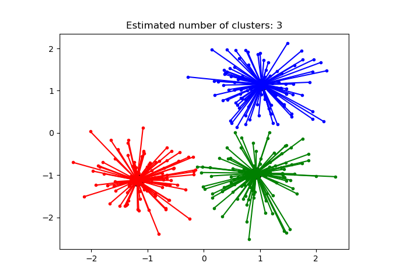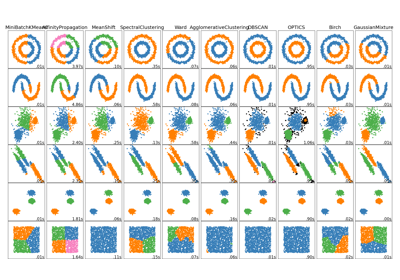# sklearn.cluster.AffinityPropagation¶

class sklearn.cluster.AffinityPropagation(*, damping=0.5, max_iter=200, convergence_iter=15, copy=True, preference=None, affinity='euclidean', verbose=False, random_state='warn')[source]

Perform Affinity Propagation Clustering of data.

Read more in the User Guide.

Parameters
dampingfloat, default=0.5

Damping factor (between 0.5 and 1) is the extent to which the current value is maintained relative to incoming values (weighted 1 - damping). This in order to avoid numerical oscillations when updating these values (messages).

max_iterint, default=200

Maximum number of iterations.

convergence_iterint, default=15

Number of iterations with no change in the number of estimated clusters that stops the convergence.

copybool, default=True

Make a copy of input data.

preferencearray-like of shape (n_samples,) or float, default=None

Preferences for each point - points with larger values of preferences are more likely to be chosen as exemplars. The number of exemplars, ie of clusters, is influenced by the input preferences value. If the preferences are not passed as arguments, they will be set to the median of the input similarities.

affinity{‘euclidean’, ‘precomputed’}, default=’euclidean’

Which affinity to use. At the moment ‘precomputed’ and euclidean are supported. ‘euclidean’ uses the negative squared euclidean distance between points.

verbosebool, default=False

Whether to be verbose.

random_stateint or np.random.RandomStateInstance, default: 0

Pseudo-random number generator to control the starting state. Use an int for reproducible results across function calls. See the Glossary.

New in version 0.23: this parameter was previously hardcoded as 0.

Attributes
cluster_centers_indices_ndarray of shape (n_clusters,)

Indices of cluster centers

cluster_centers_ndarray of shape (n_clusters, n_features)

Cluster centers (if affinity != precomputed).

labels_ndarray of shape (n_samples,)

Labels of each point

affinity_matrix_ndarray of shape (n_samples, n_samples)

Stores the affinity matrix used in fit.

n_iter_int

Number of iterations taken to converge.

Notes

For an example, see examples/cluster/plot_affinity_propagation.py.

The algorithmic complexity of affinity propagation is quadratic in the number of points.

When fit does not converge, cluster_centers_ becomes an empty array and all training samples will be labelled as -1. In addition, predict will then label every sample as -1.

When all training samples have equal similarities and equal preferences, the assignment of cluster centers and labels depends on the preference. If the preference is smaller than the similarities, fit will result in a single cluster center and label 0 for every sample. Otherwise, every training sample becomes its own cluster center and is assigned a unique label.

References

Brendan J. Frey and Delbert Dueck, “Clustering by Passing Messages Between Data Points”, Science Feb. 2007

Examples

>>> from sklearn.cluster import AffinityPropagation
>>> import numpy as np
>>> X = np.array([[1, 2], [1, 4], [1, 0],
...               [4, 2], [4, 4], [4, 0]])
>>> clustering = AffinityPropagation(random_state=5).fit(X)
>>> clustering
AffinityPropagation(random_state=5)
>>> clustering.labels_
array([0, 0, 0, 1, 1, 1])
>>> clustering.predict([[0, 0], [4, 4]])
array([0, 1])
>>> clustering.cluster_centers_
array([[1, 2],
[4, 2]])


Methods

 fit(X[, y]) Fit the clustering from features, or affinity matrix. fit_predict(X[, y]) Fit the clustering from features or affinity matrix, and return cluster labels. get_params([deep]) Get parameters for this estimator. Predict the closest cluster each sample in X belongs to. set_params(**params) Set the parameters of this estimator.
__init__(*, damping=0.5, max_iter=200, convergence_iter=15, copy=True, preference=None, affinity='euclidean', verbose=False, random_state='warn')[source]

Initialize self. See help(type(self)) for accurate signature.

fit(X, y=None)[source]

Fit the clustering from features, or affinity matrix.

Parameters
Xarray-like or sparse matrix, shape (n_samples, n_features), or array-like, shape (n_samples, n_samples)

Training instances to cluster, or similarities / affinities between instances if affinity='precomputed'. If a sparse feature matrix is provided, it will be converted into a sparse csr_matrix.

yIgnored

Not used, present here for API consistency by convention.

Returns
self
fit_predict(X, y=None)[source]

Fit the clustering from features or affinity matrix, and return cluster labels.

Parameters
Xarray-like or sparse matrix, shape (n_samples, n_features), or array-like, shape (n_samples, n_samples)

Training instances to cluster, or similarities / affinities between instances if affinity='precomputed'. If a sparse feature matrix is provided, it will be converted into a sparse csr_matrix.

yIgnored

Not used, present here for API consistency by convention.

Returns
labelsndarray, shape (n_samples,)

Cluster labels.

get_params(deep=True)[source]

Get parameters for this estimator.

Parameters
deepbool, default=True

If True, will return the parameters for this estimator and contained subobjects that are estimators.

Returns
paramsmapping of string to any

Parameter names mapped to their values.

predict(X)[source]

Predict the closest cluster each sample in X belongs to.

Parameters
Xarray-like or sparse matrix, shape (n_samples, n_features)

New data to predict. If a sparse matrix is provided, it will be converted into a sparse csr_matrix.

Returns
labelsndarray, shape (n_samples,)

Cluster labels.

set_params(**params)[source]

Set the parameters of this estimator.

The method works on simple estimators as well as on nested objects (such as pipelines). The latter have parameters of the form <component>__<parameter> so that it’s possible to update each component of a nested object.

Parameters
**paramsdict

Estimator parameters.

Returns
selfobject

Estimator instance.

## Examples using sklearn.cluster.AffinityPropagation¶